# Time Complexity MCQ - 2

## 15 Questions MCQ Test RRB JE for Computer Science Engineering | Time Complexity MCQ - 2

Description
This mock test of Time Complexity MCQ - 2 for Computer Science Engineering (CSE) helps you for every Computer Science Engineering (CSE) entrance exam. This contains 15 Multiple Choice Questions for Computer Science Engineering (CSE) Time Complexity MCQ - 2 (mcq) to study with solutions a complete question bank. The solved questions answers in this Time Complexity MCQ - 2 quiz give you a good mix of easy questions and tough questions. Computer Science Engineering (CSE) students definitely take this Time Complexity MCQ - 2 exercise for a better result in the exam. You can find other Time Complexity MCQ - 2 extra questions, long questions & short questions for Computer Science Engineering (CSE) on EduRev as well by searching above.
QUESTION: 1

### Consider the following segment of C-code: int j, n; j = 1; while (j <= n)     j = j * 2;

Solution: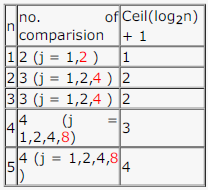may be we have to count those comparisons which results in the execution of loop.

Answer should be Ceil(log2n) + 1
EDIT: but answer could be: floor(log2n) + 2

QUESTION: 2

### In the following C function, let n ≥ m int gcd(n,m) { if (n%m == 0) return m;     n = n%m; return gcd(m,n); } How many recursive calls are made by this function?

Solution:

Worst case will arise when both n and m are consecutive Fibonacci numbers.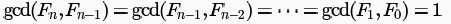and nth Fibonacci number is 1.618n  where 1.618 is the Golden ratio.

So, to find gcd(n,m), number of recursive calls will be θ(logn)

QUESTION: 3

### Consider the following C program segment: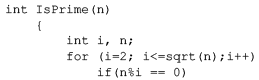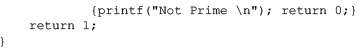Let T(n) denote number of times the for loop is executed by the program on input . Which of the following is TRUE?

Solution:

Worst Case :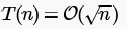Best Case : When n is an even number body of for loop is executed only 1 time (due to "return 0" inside if) which is  irrespective of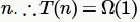QUESTION: 4

Exponentiation is a heavily used operation in public key cryptography. Which of the following options is the tightest upper bound on the number of multiplications required to compute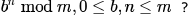Solution:

We need to divide
n recursively and compute like following: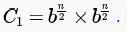In this, we need to calculate bn/2 only once.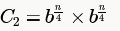.

.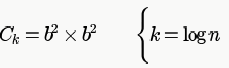Recurrence relation: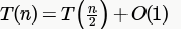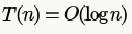QUESTION: 5

Let P1,P2,..... Pn points in the xy-plane such that no three of them are collinear. For every pair of points Pi and Pj, Let Lij be the line passing through them. Let Lab  be the line with the steepest gradient among all n(n-1)/2 lines. The time complexity of the best algorithm for finding  Pa and Pb is

Solution:

For gradient to be maximum x2-x1 should be minimum. So, sort the points (in θ(nlogn) time) according to x coordinate and find the minimum difference between them (in θ(n) time).

Best complexity: θ(nlogn + n) which leads to B.

QUESTION: 6

The minimum number of comparisons required to determine if an integer appears more than n/2 times in a sorted array of n integers is

Solution:

whenever there exists an element which is present in the array : more than n/2 times, then definitely it will be present at the middle index position; in addition to that it will also be present at anyone of the neighbourhood indices namely i - 1 and i + 1

No matter how we push that stream of More than n/2 times of elements of same value around the Sorted Array, it is bound to be present at the middle index + atleast anyone of its neighbourhood once we got the element which should have occurred more that n/2 times.we count its total occurrences in O(logn) time.

To check whether a given number is repeated n/2 times in the array can be done in O(log n) time.

Algo

1. find the first occurrence (index i) of x(given number) in the array which can be done in O(log n) time (a variant of binary search).

2. check if A[i] == A[n/2+i]

return true
3. else return false

QUESTION: 7

Consider the following pseudo code. What is the total number of multiplications to be performed?

D = 2
for i = 1 to n do

for j = i to n do

for k = j + 1 to n do

D = D * 3

Solution:

Total number of multiplications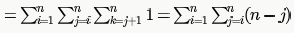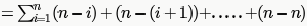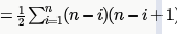QUESTION: 8

An algorithm performs   (log N)1/2   find operations , N insert operations, (log N)1/2 delete operations, and (log N)1/2 decreasekey operations on a set of data items with keys drawn from a linearly ordered set . For a delete operation, a pointer is provided to the record that must be deleted . For the decrease-key operation, a pointer is provided to the record that has its key decreased. Which one of the following data structures is the most suited for the algorithm to use, if the goal is to achieve the best total asymptotic complexity considering all the operations?

Solution:

The operations given can be performed in any order. So, for Min-heap we cannot do the usual BuildHeap method.

Delete in unsorted array is O(1) as we can just swap the deleted element with the last element in the array and delete the last element.

For sorted-doubly linked-list we cannot do binary search as this would require another array to maintain the pointers to the
nodes.

QUESTION: 9

Two main measures for the efficiency of an algorithm are

Solution:
QUESTION: 10

Match the algorithms with their time complexities: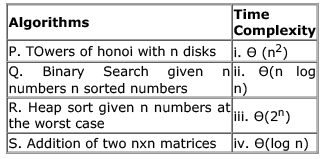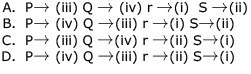Solution:

According to the recurrrence relation

T(n) = 2 T( n-1 ) +1

Tower of hanoi we get it is Ɵ(2n)
now heap sort worst case Ɵ(n log n)

Binary Search given n numbers n sorted numbers Ɵ(log n)
Addition of two nxn matrices Ɵ (n2)
so C is correct answer here

QUESTION: 11

Consider the following C function

int fun(int n) {

int I, j;

for(i=1; i<=n; i++) {

for (j=1; j<n; j+=i) {

printf(“%d %d”, I, j);

}
}
}

Time complexity of fun in terms of θ notation is

Solution:

inner for loop is dependent on i, so for each i we have to check no of times inner loop operating..

it ll be something like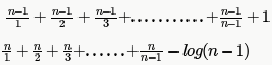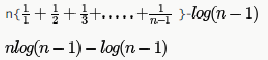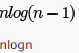QUESTION: 12

It takes O(n) time to find the median in a list of n elements, which are not necessarily in sorted order while it takes only  O(1) time to find the median in a list of n sorted elements. How much time does it take to find the median of 2n elements. which are given as two lists of  sorted elements each?

Solution:

1) Calculate the medians m1 and m2 of the input arrays ar1[]
and ar2[] respectively.
2) If m1 and m2 both are equal.
return m1 (or m2)
3) If m1 is greater than m2, then median is present in one
of the below two subarrays.
a) From first element of ar1 to m1 (ar1[0 to n/2])
b) From m2 to last element of ar2 (ar2[n/2 to n-1])
4) If m2 is greater than m1, then median is present in one
of the below two subarrays.
a) From m1 to last element of ar1 (ar1[n/2 to n-1])
b) From first element of ar2 to m2 (ar2[0 to n/2])
5) Repeat the above process until size of both the subarrays
becomes 2.

6) If size of the two arrays is 2 then
the median.
Median = (max(ar1, ar2) + min(ar1, ar2))/2
Time complexity O(logn)

QUESTION: 13

Which of the following statements is TRUE for all sufficiently large n?

Solution:

Let us take log for each function.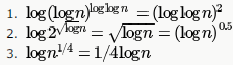Here, If we consider logn as a term (which is common in all 3), first 1 is a log function, second one is sqrt function and third one is linear function of logn . Order of growth of these functions are well known and log is the slowest growing followed by sqrt and then linear. So, option A is the correct answer here.

PS: After taking log is we arrive at functions distinguished by some constant terms only, then we can not conclude the order of grpwth of the original functions using the log function. Examples are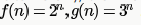QUESTION: 14

Consider the following code fragment in the C programming language when run on a non-negative integer n.

int f (int n)

{
if (n==0 || n==1)

return 1;

else

return f (n - 1) + f(n - 2);
}

Assuming a typical implementation of the language, what is the running time of this algorithm and how does it compare to the optimal running time for this problem?

Solution:

it is fibanacci series generation. it takes exponential time if we won't use dynamic programming.
if we use dynamic programming then it takes O(n)

QUESTION: 15

Two alternative packages A and B are available for processing a database having 10k records. Package A requires  0.0001n2 time units and package  B requires  10nlog10ntime units to process n records. What is the smallest value of k for which package B will be preferred over A?

Solution: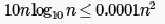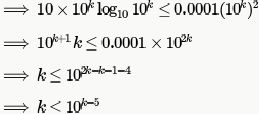Trying the values, 5 doesn't satisfy this but 6 satisfies.